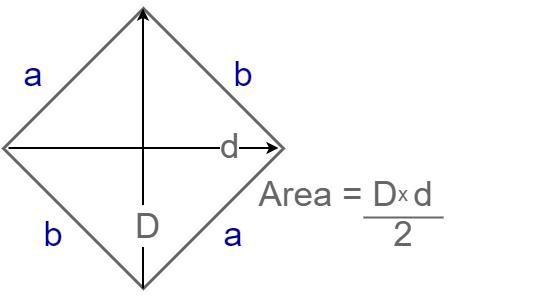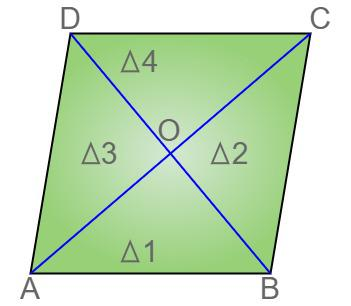Open in App
Not now

# Area of a Rhombus

• Last Updated : 25 Aug, 2022

Rhombus is a special type of Parallelogram which is of huge importance. In a rhombus, all four sides are equal and opposite pairs of lines are congruent, also opposite angles in a rhombus are equal. Rhombus often gets confused with square but Rhombus is very different from the square. Why a rhombus is not considered a square is explained here. Area of the Rhombus is the space occupied by the boundaries of the Rhombus in 2-D space. Area of the Rhombus can be calculated using various methods some of which are discussed in this article.

## What is Area of a Rhombus?

Area of the rhombus is considered as the space enclosed by the Rhombus in the 2-D plane. It depends on the dimensions of the rhombus. It is measured in square units, such as square meters, square centimeters, etc. Area of Rhombus is measured using the formulas listed below

## Area of Rhombus Formula

Area of the rhombus can be found using various methods some of them are listed in the table below

Where,

D = length of first diagonal
d = length of second diagonal
b = length of side of rhombus
h = height of rhombus
a = measure of an interior angle## Derivation of Area Formula for Rhombus

Let us consider a rhombus ABCD with O as the point of intersection of two diagonals AC and BD.The area of rhombus will be

Area = 4 × area of △AOB

= 4 × (1/2) × AO × OB sq.units

= 4 × (1/2) × (1/2) d1 × (1/2) d2 sq.unit

= 4 × (1/8) d1 × d2

= 1/2 d1 × d2

Therefore, the area of a rhombus is A = 1/2 d1 × d2.

## How to Find the Area of a Rhombus?

The area of a rhombus is the total space covered or enclosed by the rhombus on a two-dimensional plane. The area of the rhombus can be calculated by three different methods by using diagonal, using base and height, and using trigonometry.

Different methods for finding area of Rhombus are given below

### Area of Rhombus using Diagonal

Area = (d1 × d2)/2 sq. units

Where,

d1 is the length of diagonal 1

d2  is the length of diagonal 2

Example 1: Find the area of a rhombus having diagonals 16 m and 18 m.

Solution:

Diagonal 1, d1 = 16 m

Diagonal 2, d2 = 18 m

Area of a rhombus, A = (d1 × d2) / 2

= (16 × 18) / 2

= 288 / 2

= 144 m2

Thus, the area of the rhombus is 144 m2

### Area of Rhombus using Base and Height

Area of a Rhombus = b × h sq units

Where,

b is the length of any side of the rhombus

h is the height of the rhombus

Example 2: Find the area of a rhombus having base of 12 m and height is 16 m.

Solution:

Base, b = 12 m

Height, h = 16 m

Area, A = b × h

= 12 × 16 m2

A = 192 m2

Thus, the area of the rhombus is 192 m2

### Area of Rhombus using Trigonometric Ratios

Area of a Rhombus = b2 × sin(A) sq. units

Where,

b is the length of any side of the rhombus

A is a measure of any interior angle

Example 3: Find the area of a rhombus if the length of its side is 12 m and one of its angles A is 60°

Solution:

Side = s = 12 m

Angle A = 60°

Area = s2 × sin (60°)

A = 144 × √3/2

A = 72√3 m2

## Solved Examples on Area of Rhombus

Example 1: Calculate the area of a rhombus (using base and height) if its base is 5cm and height is 3cm.

Solution:

Given,

Base (b) = 5cm

height of rhombus(h) = 3cm

Now,’

Area of the rhombus(A) = b × h

= 5 × 3

= 15cm2

Example 2: Calculate the area of a rhombus (using diagonal) having diagonals equal to 4cm and 3cm.

Solution:

Given,

Length of diagonal 1 (d1) = 4cm

Length of diagonal 2 (d2) = 3cm

Now,

Area of Rhombus (A) = 1/2 d1 × d2

= 4 x3/2 = 6cm2

Example 3: Calculate the area of the rhombus (using trigonometry) if its side is 8cm and one of its angles A is 30 degrees.

Solution:

Side of the rhombus (b) = 8cm

angle (a) = 30 degrees

Now,

Area of the rhombus(A) = b2 × sin(a)

= (8) × sin(30)

= 64 × 1/2 = 32 cm2

Example 4: Calculate the base of a rhombus if its area is  25cm2 and height is 10cm.

Solution:

Given,

Area = 25 cm2

height of rhombus(h) = 10 cm

Now,

Area of the rhombus(A) = b × h

25  = b × 10

= 2.5 cm

## FAQs on Area of Rhombus

Question 1: What do we mean by Rhombus?

A rhombus is a type of quadrilateral whose opposite sides are parallel and equal. Also, the opposite angles of a rhombus are equal and the diagonals bisect each other at right angles.

Question 2: Give the Formula for finding the Area of a Rhombus.

For finding the area of a rhombus, the given formula is used:

A = ½ × d1 × d2

where, d1 and d2 are diagonals of rhombus

Question 3: How to calculate the perimeter of a rhombus?

Solution:

The perimeter of a rhombus can be calculated by the formula

P= 4b units

where b is a side of the rhombus.

Question 4: How to Find the Area of a Rhombus When the Side and Height are Given?

The area of a rhombus its height and side are given is calculated using

A = Base × Height sq units

Question 5: Can area of a rhombus be same as the area of a square?